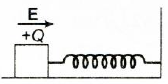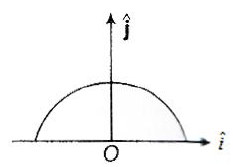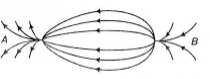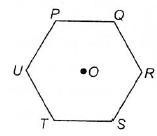Courses

# Electric Field Practice Level - 1

## 25 Questions MCQ Test | Electric Field Practice Level - 1

Description
This mock test of Electric Field Practice Level - 1 for Class 12 helps you for every Class 12 entrance exam. This contains 25 Multiple Choice Questions for Class 12 Electric Field Practice Level - 1 (mcq) to study with solutions a complete question bank. The solved questions answers in this Electric Field Practice Level - 1 quiz give you a good mix of easy questions and tough questions. Class 12 students definitely take this Electric Field Practice Level - 1 exercise for a better result in the exam. You can find other Electric Field Practice Level - 1 extra questions, long questions & short questions for Class 12 on EduRev as well by searching above.
QUESTION: 1

### A wooden block performs SHM on a frictionless surface with frequency v0. The block carries a charge + Q on its surface. If now a uniform electric field E is switched on as shown, then the SHM of the block will beSolution:
*Multiple options can be correct
QUESTION: 2

Solution:
QUESTION: 3

### A thin semi-circular ring of radius r has a positive charge q distributed uniformly over it. The net field E at the centre O isSolution:
QUESTION: 4

An electron initially at rest falls a distance of 1.5 cm in a uniform electric field of magnitude 2 × 104 N/C. The time taken by the electron to fall this distance is

Solution:
QUESTION: 5

The electric field created by a point charge falls with distance r from the point charge as

Solution:
QUESTION: 6

The electric field at the centroid of an equilateral triangle carrying an equal charge q at each of the vertices is

Solution:
QUESTION: 7

A particle of mass m carrying charge q is kept at rest in a uniform electric field E and then released. The kinetic energy gained by the particle, when it moves through a distance y is

Solution:
QUESTION: 8

The charge q is projected into a uniform electric field E, work done when it moves a distance Y is

Solution:
QUESTION: 9

If the linear charge density of a cylinder is 4 μCm-1 then electric field intensity at point 3.6 cm from axis is

Solution:
QUESTION: 10

A simple pendulum has a length l and the mass of the bob is m. The bob is given a charge q coulomb. The pendulum is suspended between the vertical plates of a charged parallel plate capacitor. If E is the electric field strength between the plates, the time period of the pendulum is given by

Solution:
QUESTION: 11

Charges +2q +q and +q are placed at the corners A, B and C of an equilateral triangle ABC. If E is the electric field at the circumcentre O of the triangle, due to the charge +q, then the magnitude and direction of the resulatant electric field at O is

Solution:
QUESTION: 12

Which of the following configurations of electric lines of force is not possible?

Solution:
QUESTION: 13

Two unlike charges of the same magnitude Q are placed at a distance d. The intensity of the electric field at the middle point in the line joining the two chages.

Solution:
QUESTION: 14

The spatial distribution of the electric field due to charges (A, B) is shown in figure. Which one of the following statements is correct?Solution:
QUESTION: 15

Two point charges +8q and -2 q are located at x = 0 and x = L respectively, The location of a point on the x-axis at which the net electric field due to these two point charges is zero is

Solution:
QUESTION: 16

Six charges, three positive and three negative of equal magnitude are to be placed at the vertices of a regular hexagon such that the electric field at O is double the electric field when only one positive charge of same magnitude is placed at R. Which of the following arrangements of charges is possible for P, Q, R, S, T and U respectively?Solution:
QUESTION: 17

The electric field at a point due to an electric dipole, on an axis inclined at an angle θ (< 900) to the dipole axis, is perpendicular to the dipole axis, if the angle θ  is

Solution:
QUESTION: 18

A dipole of electric dipole moment p is placed in a uniform electric field of strength E. If θ  is the angle between positive directions of p and E, then the potential energy of the electric dipole is largest when θ is

Solution:
QUESTION: 19

Let Ea be the electric field due to a dipole in its axial plane distancei and let Eq be the field in the equatorial plane distancei ,then the relation between Ea and Eq will be

Solution:
QUESTION: 20

The relation between the intensity of the electric field of an electric dipole at a distanec r from its centre on its axis and the distance is when (r >> 2t)

Solution:
QUESTION: 21

An electric dipole of the dipole moment p is placed in a uniform electric field E. The maximum torque experienced by the dipole is

Solution:
QUESTION: 22

Torque acting on an electric dipole in a uniform electric field is maximum if the angle between p and E is

Solution:
QUESTION: 23

An electric dopole of length 1 cm is placed with the axis making an angle of 300 to an electric field of strength 104NC-1. If it experiences a torque of 10√2 Nm, the potential energy of the dipole is

Solution:
QUESTION: 24

If the force exerted by an electric dipole on a charge q at a distance of 1 m is F, the force at a point 2 m away in the same direction will be

Solution:
QUESTION: 25

Dipole is placed parallel to the electric field. If Q is the work done in rotating the dipole by 600, then work done in rotating it by 1800 is

Solution: